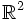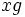# Left coset of a subgroup

(Redirected from Left coset space)
VIEW: Definitions built on this | Facts about this: (facts closely related to Left coset of a subgroup, all facts related to Left coset of a subgroup) |Survey articles about this | Survey articles about definitions built on this
VIEW RELATED: Analogues of this | Variations of this | Opposites of this |[SHOW MORE]

## Definition

### Definition with symbols

Let$H$ be a subgroup of a group$G$. Then, a left coset of$H$ is a nonempty set$S \subset G$ satisfying the following equivalent properties:

1.$x^{-1}y$ is in$H$ for any$x$ and$y$ in$S$, and for any fixed$x \in S$, the map$y \mapsto x^{-1}y$ is a surjection from$S$ to$H$
2. There exists an$x$ in$G$ such that$S = xH$ (here$xH$ is the set of all$xh$ with$h \in H$)
3. For any$x$ in$S$,$S = xH$
4.$S$ is one of the orbits in$G$ under the right action of$H$, i.e. the action of$H$ by right multiplication on$G$.

Any element$x \in S$ is termed a coset representative for$S$.

Note that$H$ is itself a left coset for$H$, and we can take as coset representative, any element of$H$ (a typical choice would be to take the identity element).

### Equivalence of definitions

For full proof, refer: Equivalence of definitions of left coset

## Examples

### Extreme examples

1. If we consider a group as a subgroup of itself, then there's only one left coset: the subgroup itself.
2. The left cosets of the trivial subgroup in a group are precisely the singleton subsets (i.e. the subsets of size one). In other words, every element forms a coset by itself.

### Examples in abelian groups

Note that for abelian groups, since multiplication is commutative, we can drop the left adjective from left cosets. Further, if we use additive notation, the coset of$g$ for a subgroup$H$ is written as$g + H$.

1. In the group of integers under addition, the left cosets of the subgroup of multiples of$n$ are the congruence classes mod$n$ (i.e. the collections of numbers that leave the same remainder mod$n$). For instance, the subgroup of even numbers in the group of integers has two left cosets: the even numbers and odd numbers (coset representatives are 0 and 1 respectively). The subgroup of multiples of 3 has three cosets: the multiples of 3, the numbers that are 1 mod 3, and the numbers that are 2 mod 3. The coset representatives can be taken to be 0,1, and 2 respectively.
2. In the group of rational numbers under addition, the subgroup of integers have, as left cosets, the collections of rational numbers having the same fractional part. The coset representative for a particular coset can be chosen as the unique element in that coset that is in the interval$[0,1)$.
3. In a vector space over a field, vector subspaces are examples of subgroups. The cosets of a vector subspace are the "parallel" affine subspaces obtained by translating it. For instance, the two-dimensional vector space$\R^2$, which can be identified with the Euclidean plane, the one-dimensional subspaces are lines through the origin. The cosets of any such one-dimensional subspace are precisely the lines parallel to the given line.

### Examples in non-Abelian groups

1. In the symmetric group on three elements on elements$1,2,3$, any subgroup of order two, say, that obtained by taking the transposition of$1$ and$2$, has three left cosets. Each coset is described by where it sends the element$3$.
2. More generally, in the symmetric group acting on elements$1,2,3,\ldots,n$, the subgroup of permutations that fix the element$n$ has exactly$n$ left cosets: the cosets are parametrized by where they send the element$n$.

## Facts

### For a single subgroup in a group

Statement Key related facts/terminology Explanation
left cosets partition a group the notion of left congruence induced by a subgroup The left cosets of a subgroup are pairwise disjoint and hence partition the group.
every subgroup has a left transversal left transversal of a subgroup -- a collection of representatives, one from each left coset of a subgroup Assuming the axiom of choice, we can pick one element from each left coset to obtain a left transversal.
left cosets are in bijection via left multiplication group acts on left coset space of subgroup by left multiplication given any two left cosets of a subgroup, there is a bijection between them via left multiplication by some element of the group.
left and right coset spaces are naturally isomorphic notion of right coset, every group is naturally isomorphic to its opposite group Via the inverse map, we can send each left coset of a subgroup to a right coset ,and vice versa.
equivalence of definitions of coset right coset of a subgroup a subset of a group occurs as the left coset of a subgroup if and only if it occurs as the right coset of a (possibly different) subgroup.
group acts on left coset space of subgroup by left multiplication If$H \le G$, then$G$ acts on the left coset space$G/H$ (i.e., the set of left cosets of$H$ in$G$) by left multiplication. This action is a transitive action.
fundamental theorem of group actions group acts on left coset space of subgroup by left multiplication In fact, the fundamental theorem of group actions shows that any transitive action of a group on a set looks like the action of a group on the left coset space of a subgroup by left multiplication.

### Relations between subgroups and relations between their cosets

Statement Explanation
coset containment implies subgroup containment If$H,K$ are subgroups of$G$ and a left coset of$H$ is contained in a left coset of$K$, then$H$ is contained in$K$.
nonempty intersection of cosets is coset of intersection The intersection of a bunch of left cosets of (possibly different) subgroups, if nonempty, is a left coset of the intersection of the subgroups.
Lattice of subgroups embeds in partition lattice Since left cosets partition a group, every subgroup defines a partition of the group. This gives an injective lattice homomorphism from the lattice of subgroups to the partition lattice of the group.
Product formula For subgroups$H,K$ of$G$, there is a natural bijection between the coset space$HK/K$ and the coset space$H/(H \cap K)$.

## Numerical facts

### Size of each left coset

Let$H$ be a subgroup of$G$ and$x$ be any element of$G$. Then, the map sending$g$ in$H$ to$xg$ is a bijection from$H$ to$xH$.

For full proof, refer: Left cosets are in bijection via left multiplication

### Number of left cosets

The number of left cosets of a subgroup is termed the index of that subgroup.

Since all left cosets have the same size as the subgroup, we have a formula for the index of the subgroup when the whole group is finite: it is the ratio of the order of the group to the order of the subgroup.

This incidentally also proves Lagrange's theorem -- the order of any subgroup of a finite group divides the order of the whole group.